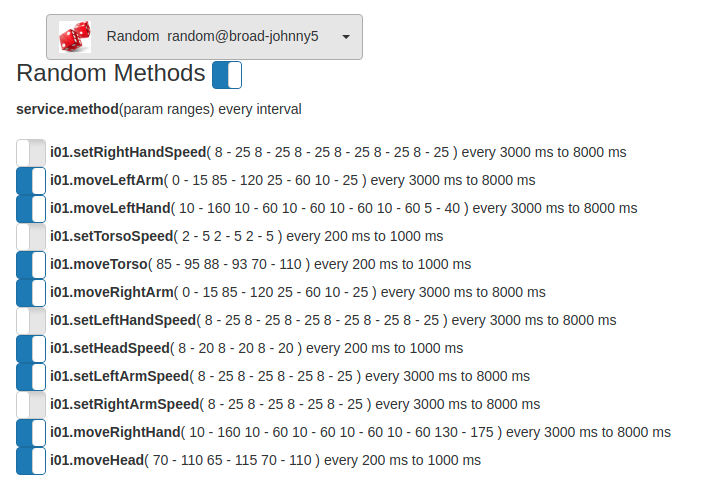Random

The Random service can create random messages with random parameters.  Any method accessable can be called at some interval with ranges of parameters.  For example, if you wanted a Servo to move at some interval between 1 and 5 seconds between 35 and 165.

random = Runtime.start('random','Random')
random.addRandom(1000, 5000, 'servo01', 'moveTo', random.intRange(35, 165))

Example of Web UI list of current random method eventsThe top switch can disable all random methods or each individual method cand be enabled or disabled.

Example for develop:

#########################################
# Random.py
# description: Random message generator - sends messages
# at random intervales with random parameters
# categories: general
#########################################

# start the service
python = Runtime.start("python","Python")
random = Runtime.start("random","Random")
clock = Runtime.start("clock","Clock")

# enable random events
random.enable()
# roll the dice every 1 to 2 seonds
random.addRandom(1000, 2000, "python", "roll_dice", random.intRange(1, 6))
def roll_dice(value):
print("roll_dice " + str(value))

random.addRandom(1000, 2000, "python", "roll_complex_dice", random.doubleRange(1, 6))
def roll_complex_dice(value):
print("roll_complex_dice " + str(value))

# roll the dice every 1 to 2 seonds
def random_color(value):
print("random_color " + str(value))

# do a complex multi parameter, multi-type method
random.addRandom(1000, 2000, "python", "kitchen_sink", random.intRange(1, 6), random.doubleRange(1, 6), random.setRange("red","green","blue","yellow"), random.setRange("bob","jane","fred","mary"))
def kitchen_sink(dice, complex_dice, colors, names):
print("kitchen_sink ", dice, complex_dice, colors, names)

# set the interval on a clock between 1000 and 8000
# if you look in the UI you can see the clock interval changing
random.addRandom(200, 500, "clock", "setInterval", random.intRange(1000, 8000))

# Chaos monkey clock starting and stopping !

# run it all for 8 seconds
sleep(8)

# disable single random event generator - must be explicit with name.method key
random.disable("python.roll_dice")
sleep(8)

# you know longer should see the python.roll_dice event firing - since it was explicitly disabled

# stop events - but leave all random event data
# allows re-enabling
# random.disable()

# enable events
# random.enable()

# stop events and clear all random event ata
# random.purge()